# R Base Graphics: An Idiot's Guide

One of the most powerful functions of R is it's ability to produce a wide range of graphics to quickly and easily visualise data. Plots can be replicated, modified and even publishable with just a handful of commands.

Making the leap from chiefly graphical programmes, such as Excel and Sigmaplot. may seem tricky. However, with a basic knowledge of R, just investing a few hours could completely revolutionise your data visualisation and workflow. Trust me - it's worth it.

Last year, I presented an informal course on the basics of R Graphics University of Turku. In this blog post, I am providing some of the slides and the full code from that practical, which shows how to build different plot types using the basic (i.e. pre-installed) graphics in R, including: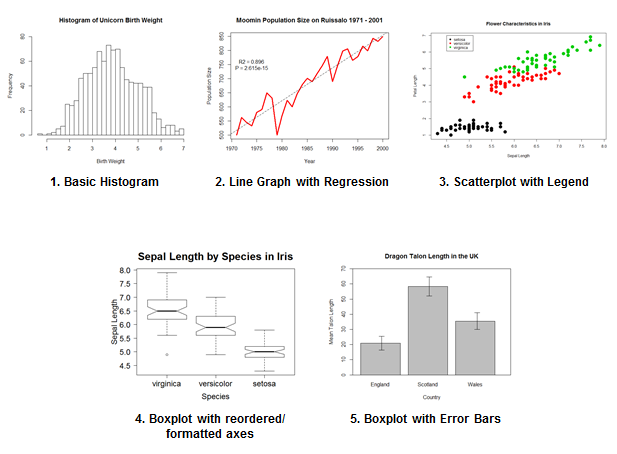Exciting, eh?

This post is BIG, but DETAILED. So, use the links below to jump ahead. I hope someone out there finds this useful - all code and datafiles are available here.

• 0. Preface: What am I supposed to know again?
• 1. Basic Histogram
• 2. Basic Line Graph with Regression
• 3. Scatterplot with Legend
• 4. Boxplot with reordered and formatted axes
• 5. Barplot with error bars
• 6. More than one plot in a window
• 7. Saving a plot

## 0. Preface: What am I supposed to know again?

Oh you. Before you get started, you should be familiar with the follow concepts:

##### Vectors!
``````height <- c(145, 167, 176, 123, 150)
weight <- c(51, 63, 64, 40, 55)

plot(height,weight)
``````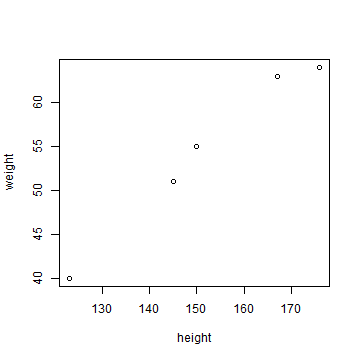#### Data frames!

``````tarsus <- read.table("tarsus.txt", header = T)
tarsus
``````
``````##    TarsusLength Weight
## 1            23    231
## 2            26    258
## 3            25    254
## 4            21    211
## 5            27    268
## 6            28    284
## 7            27    271
## 8            26    258
## 9            26    264
## 10           25    251
## 11           26    258
## 12           24    244
## 13           25    251
## 14           25    248
## 15           23    234
## 16           21    211
``````

To call a variable in the dataframe, use the \$ notation.

``````tarsus\$TarsusLength
``````
``````##   23 26 25 21 27 28 27 26 26 25 26 24 25 25 23 21
``````
``````tarsus\$Weight
``````
``````##   231 258 254 211 268 284 271 258 264 251 258 244 251 248 234 211
``````
``````
plot(tarsus\$TarsusLength,tarsus\$Weight)
``````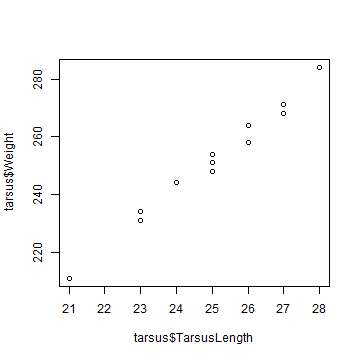#### Tables!

``````tarsus.tab <- table(tarsus\$TarsusLength)

tarsus.tab
``````
``````##
## 21 23 24 25 26 27 28
##  2  2  1  4  4  2  1
``````
``````
plot(tarsus.tab)
``````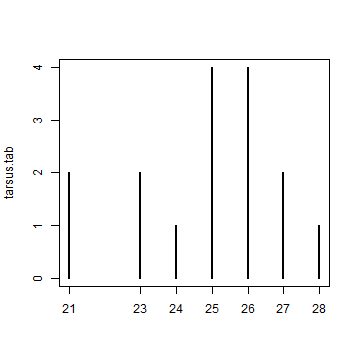``````
barplot(tarsus.tab)
``````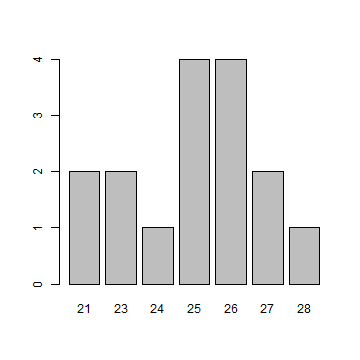## 1. Basic Histogram

What customisations are we going to learn in this section?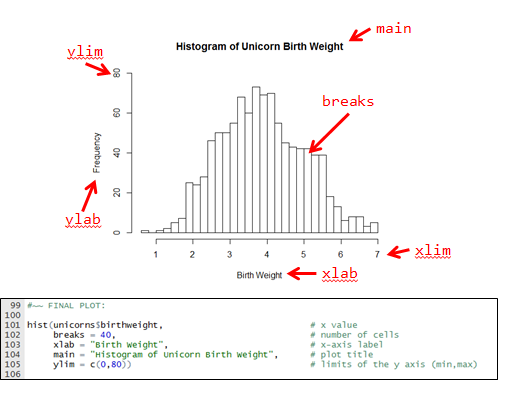Let's begin. For this part, we will use data on birthweight measured in male and female unicorns.

Let's read the data into R:

``````unicorns <- read.table("unicorns.txt" ,header = T)
``````
``````##   birthweight  sex longevity
## 1       4.478 Male         1
## 2       5.753 Male         0
## 3       3.277 Male         0
## 4       3.929 Male         0
## 5       3.973 Male         0
## 6       4.913 Male         0
``````
``````str(unicorns)
``````
``````## 'data.frame':    1000 obs. of  3 variables:
##  \$ birthweight: num  4.48 5.75 3.28 3.93 3.97 ...
##  \$ sex        : Factor w/ 2 levels "Female","Male": 2 2 2 2 2 2 2 2 2 2 ...
##  \$ longevity  : int  1 0 0 0 0 0 1 0 0 1 ...
``````

We can create a basic histogram of unicorn birthweight and longevity using hist():

``````hist(unicorns\$birthweight)            # normal distribution
``````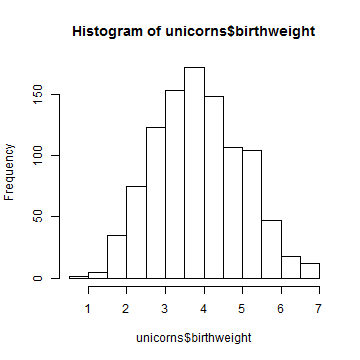``````hist(unicorns\$longevity)              # poisson distribution
``````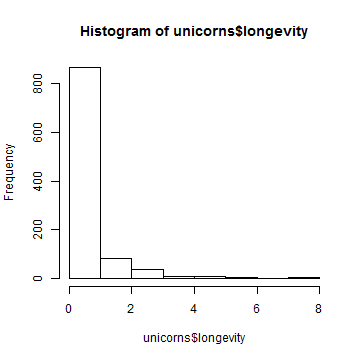And we can specify the number of cells for the histogram using: breaks = N:

``````hist(unicorns\$birthweight, breaks = 40)
``````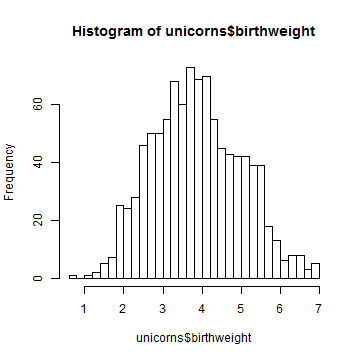``````hist(unicorns\$birthweight, breaks = c(0,1,2,3,4,5,6,7))
``````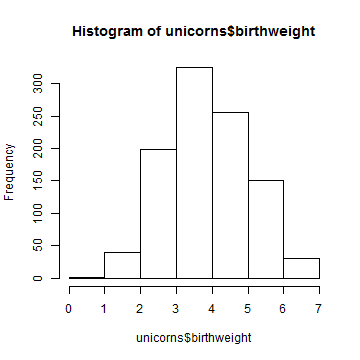``````hist(unicorns\$birthweight, breaks = c(0,1,2,3,4,7))
``````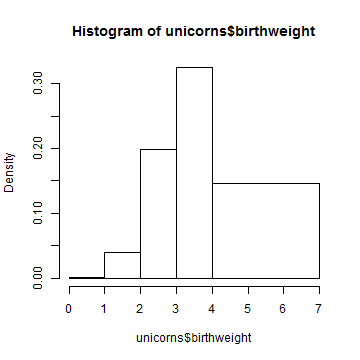Relabel the x-axis using: xlab = “Text”

``````hist(unicorns\$birthweight, breaks = 40, xlab = "Birth Weight")
``````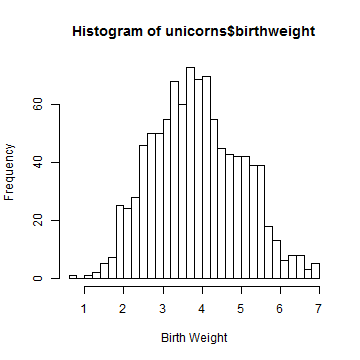Relabel the main title using: main = “Text”

``````hist(unicorns\$birthweight,
breaks = 40,
xlab = "Birth Weight",
main = "Histogram of Unicorn Birth Weight")
``````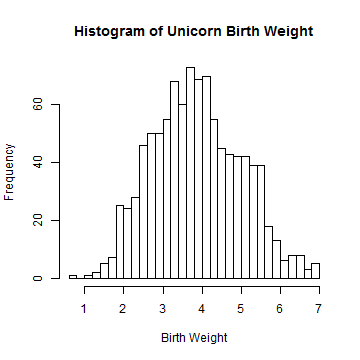NB: In our code, the lines are starting to get quite long. When there is a comma, R knows that there is more information on the next line!

The y-axis stops short of the highest value in the histogram. Lets specify new limits using: ylim = c(minimum, maximum)

``````
hist(unicorns\$birthweight,
breaks = 40,
xlab = "Birth Weight",
main = "Histogram of Unicorn Birth Weight",
ylim = c(0,80))
``````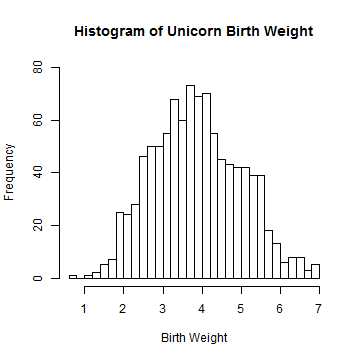## 2. Basic Line Graph with Regression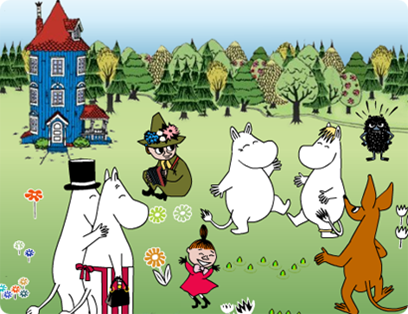Moomins are a common pest species in Finland. We have data on their population on the island of Ruissalo from 1971 to 2000.

Which customisations will we learn here?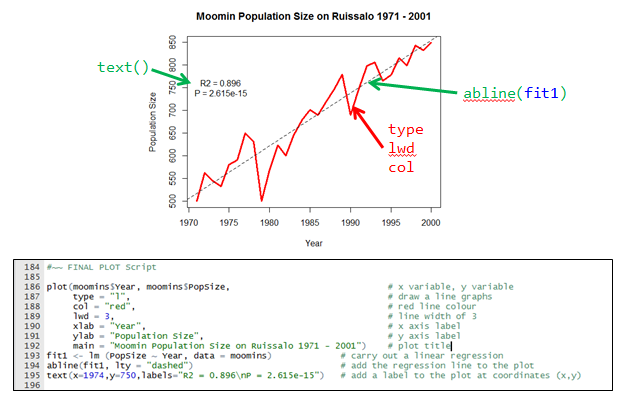``````moomins <- read.table("Moomin Density.txt", header = T)
``````
``````##   Year PopSize
## 1 1971     500
## 2 1972     562
## 3 1973     544
## 4 1974     532
## 5 1975     580
## 6 1976     590
``````

We can easily create a plot using the command `plot`.

``````plot(moomins\$Year, moomins\$PopSize)
``````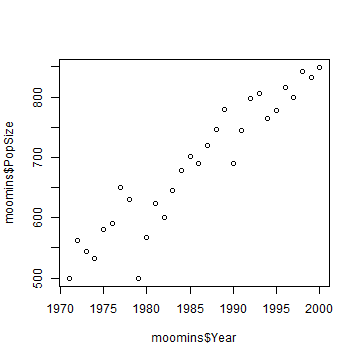There are several types of plot within the plot function. Use “type”:

``````plot(moomins\$Year, moomins\$PopSize, type = "l")     # Try "o" "p" "l" "b"
``````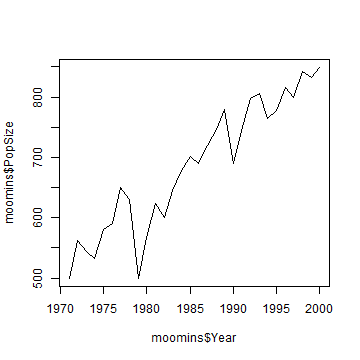We can also change the line type using “lty”

``````plot(moomins\$Year, moomins\$PopSize, type = "l", lty = "dashed")
``````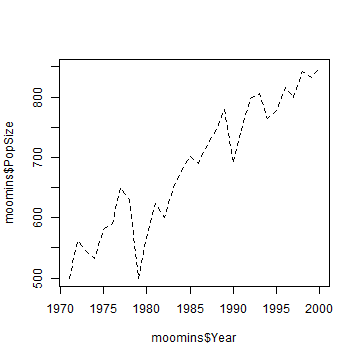``````plot(moomins\$Year, moomins\$PopSize, type = "l", lty = "dotted")
``````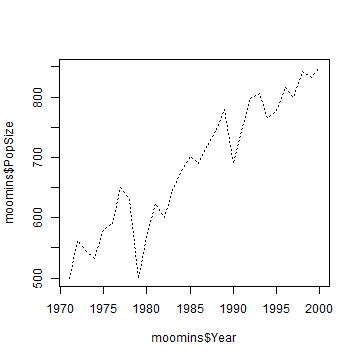The solid line looks best, so lets stick with it.

``````plot(moomins\$Year, moomins\$PopSize, type = "l")
``````Let's start to add colour using “col”.

``````plot(moomins\$Year, moomins\$PopSize, type = "l", col = "red")    # R Colour Chart
``````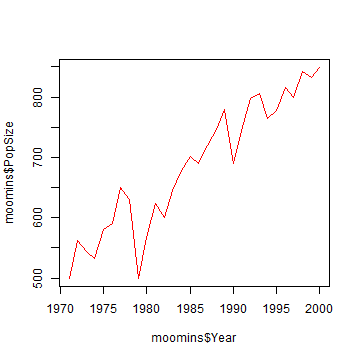NB. numbers can also be used as colours!

``````plot(moomins\$Year, moomins\$PopSize, type = "l", col = 3)
``````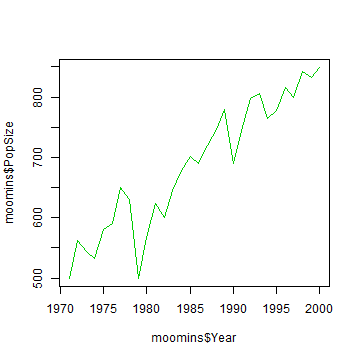Let's make the line a little thicker using “lwd” (line width)

``````plot(moomins\$Year, moomins\$PopSize, type = "l", col = "red", lwd = 3)
``````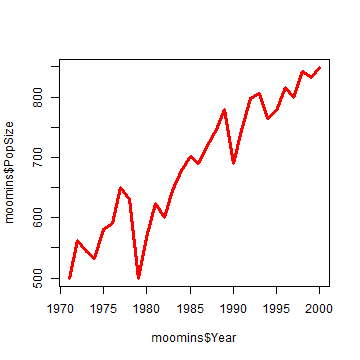Finally, lets sort out the axis titles plot title:

``````plot(moomins\$Year, moomins\$PopSize,
type = "l",
col = "red",
lwd = 3,
xlab = "Year",
ylab = "Population Size",
main = "Moomin Population Size on Ruissalo 1971 - 2001")
``````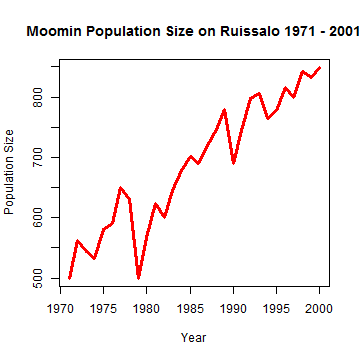Is the Moomin population increasing in size? We can add a basic linear regression to the plot using `abline`. NB. we can also use lty, lwd, col here.

``````
plot(moomins\$Year, moomins\$PopSize,
type = "l",
col = "red",
lwd = 3,
xlab = "Year",
ylab = "Population Size",
main = "Moomin Population Size on Ruissalo 1971 - 2001")

fit1 <- lm (PopSize ~ Year, data = moomins)
summary(fit1)
``````
``````##
## Call:
## lm(formula = PopSize ~ Year, data = moomins)
##
## Residuals:
##     Min      1Q  Median      3Q     Max
## -109.52  -17.76    1.65   20.37   63.83
##
## Coefficients:
##              Estimate Std. Error t value Pr(>|t|)
## (Intercept) -22493.93    1489.99   -15.1  5.6e-15 ***
## Year            11.67       0.75    15.6  2.6e-15 ***
## ---
## Signif. codes:  0 '***' 0.001 '**' 0.01 '*' 0.05 '.' 0.1 ' ' 1
##
## Residual standard error: 35.6 on 28 degrees of freedom
## Multiple R-squared:  0.896,  Adjusted R-squared:  0.893
## F-statistic:  242 on 1 and 28 DF,  p-value: 2.61e-15
``````
``````
abline(fit1, lty = "dashed")    #abline(a = intercept, b = slope)

#~~ We can add some text to the plot giving the R2 value and the P value using "text" and specifying the x and y coordinates for the text.

text(x  =1978, y = 750,labels="R2 = 0.896\nP = 2.615e-15")
``````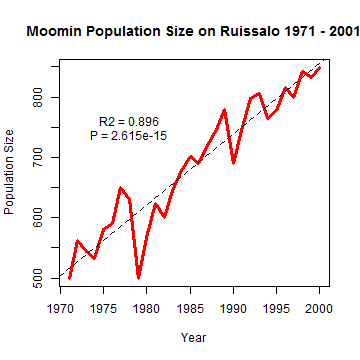Final script:

``````plot(moomins\$Year, moomins\$PopSize,                              # x variable, y variable
type = "l",                                                 # draw a line graphs
col = "red",                                                # red line colour
lwd = 3,                                                    # line width of 3
xlab = "Year",                                              # x axis label
ylab = "Population Size",                                   # y axis label
main = "Moomin Population Size on Ruissalo 1971 - 2001")    # plot title

fit1 <- lm (PopSize ~ Year, data = moomins)             # carry out a linear regression
abline(fit1, lty = "dashed")                            # add the regression line to the plot
text(x = 1978, y = 750, labels = "R2 = 0.896\nP = 2.615e-15")   # add a label to the plot at (x,y)
``````

## 3. Scatterplot with Legend

What will we learn here?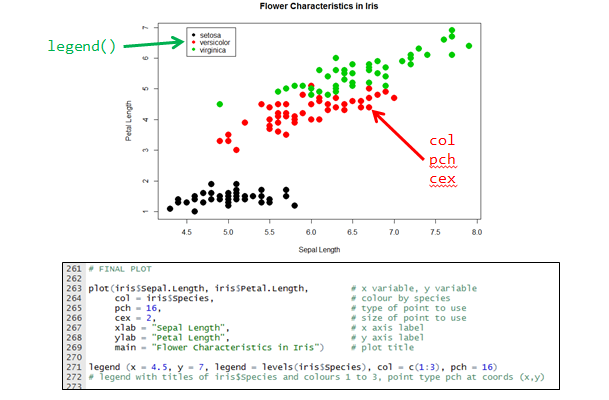R comes with many datasets preinstalled. Let's load a dataset of Flower characteristics in 3 species of Iris.

``````data(iris)
``````
``````##   Sepal.Length Sepal.Width Petal.Length Petal.Width Species
## 1          5.1         3.5          1.4         0.2  setosa
## 2          4.9         3.0          1.4         0.2  setosa
## 3          4.7         3.2          1.3         0.2  setosa
## 4          4.6         3.1          1.5         0.2  setosa
## 5          5.0         3.6          1.4         0.2  setosa
## 6          5.4         3.9          1.7         0.4  setosa
``````

There is a lot of data here! Let's explore using the 'pairs' function

``````pairs(iris)
``````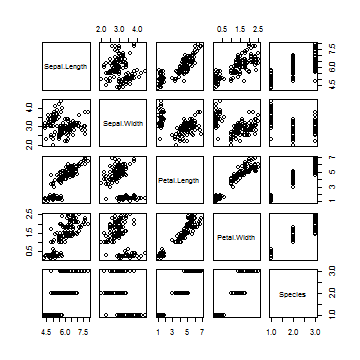This doesn't tell us much about the species differences. We can tell R to plot using a different colour for the three species of iris:

``````pairs(iris, col = iris\$Species)
``````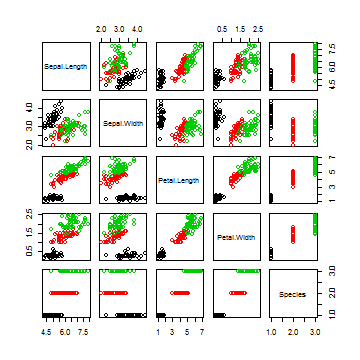Sepal.Length and Petal.Length look interesting! Let's start by looking at that. Again, we will specify colour as the Species.

``````plot(iris\$Sepal.Length, iris\$Petal.Length, col = iris\$Species)
``````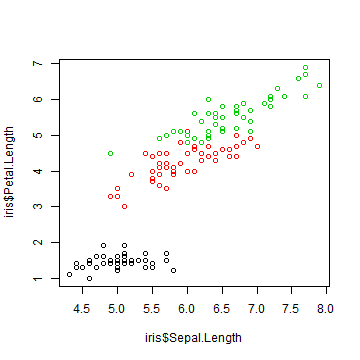These points are difficult to see! Let's pick some different ones using “pch”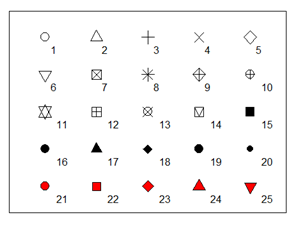``````
plot(iris\$Sepal.Length, iris\$Petal.Length, col = iris\$Species, pch = 15)
``````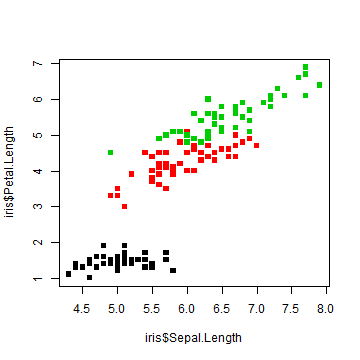``````plot(iris\$Sepal.Length, iris\$Petal.Length, col = iris\$Species, pch = "A")
``````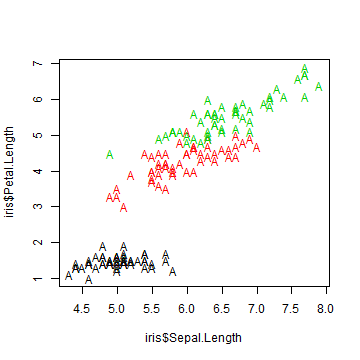pch 21:25 also specify an edge colour (col) and a background colour (bg)

``````plot(iris\$Sepal.Length, iris\$Petal.Length, col = iris\$Species, pch = 21, bg = "blue")
``````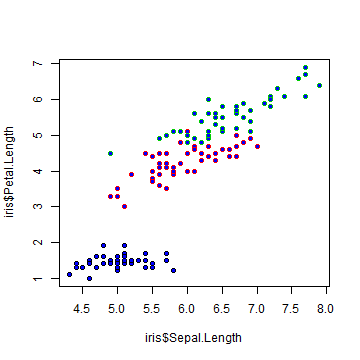lets settle on solid circles `(pch = 16)`

``````
plot(iris\$Sepal.Length, iris\$Petal.Length, col = iris\$Species, pch = 16)
``````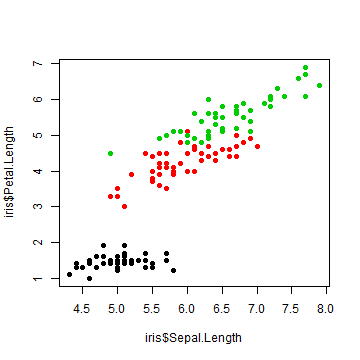We can change the size of the points with “cex”

``````plot(iris\$Sepal.Length, iris\$Petal.Length,
col = iris\$Species,
pch = 16,
cex = 2)
``````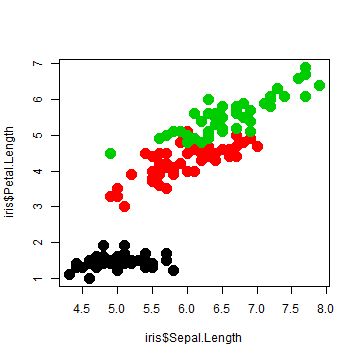It's difficult to tell these points apart, so perhaps we should make a legend. This is one of the major drawbacks with R. iris\$Species is a factor, and R will automatically order factors in alphabetical order.

``````levels(iris\$Species)
``````
``````##  "setosa"     "versicolor" "virginica"
``````

Therefore, setosa, versicolor and virginica will correspond to 1, 2 and 3 on the plot default colours. Keep this in mind for the next part!

``````plot(iris\$Sepal.Length, iris\$Petal.Length,
col = iris\$Species,
pch = 16,
cex = 2)
legend(x = 4.5, y = 7, legend = levels(iris\$Species), col = c(1:3), pch = 16)
``````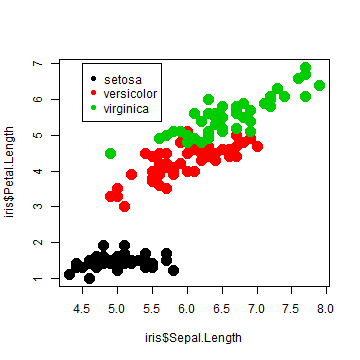FINAL PLOT

``````plot(iris\$Sepal.Length, iris\$Petal.Length,        # x variable, y variable
col = iris\$Species,                          # colour by species
pch = 16,                                    # type of point to use
cex = 2,                                     # size of point to use
xlab = "Sepal Length",                       # x axis label
ylab = "Petal Length",                       # y axis label
main = "Flower Characteristics in Iris")     # plot title

legend (x = 4.5, y = 7, legend = levels(iris\$Species), col = c(1:3), pch = 16)
``````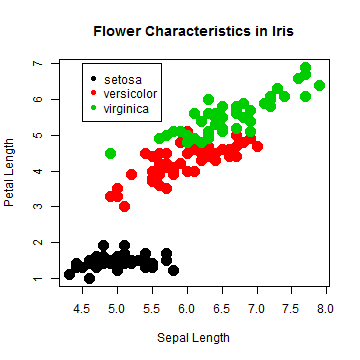``````#~~ legend with titles of iris\$Species and colours 1 to 3, point type pch at coords (x,y)
``````

SIDE NOTE 1: specifying colours: It is also possible to specify colours in your data frame.

``````iris\$Colour <- ""
iris\$Colour[iris\$Species=="setosa"] <- "magenta"
iris\$Colour[iris\$Species=="versicolor"] <- "cyan"
iris\$Colour[iris\$Species=="virginica"] <- "yellow"

plot(iris\$Sepal.Length, iris\$Petal.Length, col = iris\$Colour, pch = 16, cex = 2)
legend(x = 4.5, y = 7,
legend = c("setosa","versicolor","virginica"),
col = c("magenta","cyan","yellow"),
pch=16)
``````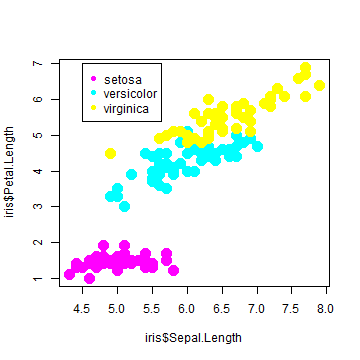SIDE NOTE 2: It would also be possible to specify lines in the legend by using “lty” instead of “pch”

``````plot(iris\$Sepal.Length, iris\$Petal.Length, col = iris\$Species, pch = 16, cex = 2)
legend(4.5, 7,
legend = c("setosa","versicolor","virginica"),
col = c(1:3),
lty = "solid")
``````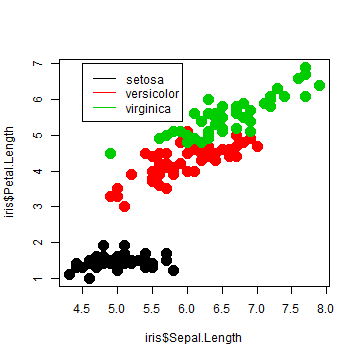## 4. Boxplot with reordered and formatted axes

What will be tackle here?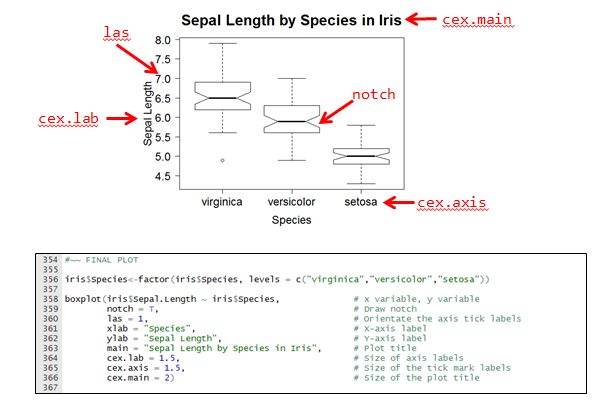We will continue to use the Iris dataset for this section. Let's examine the distribution of Sepal Length for each species:

``````
boxplot(iris\$Sepal.Length ~ iris\$Species)
``````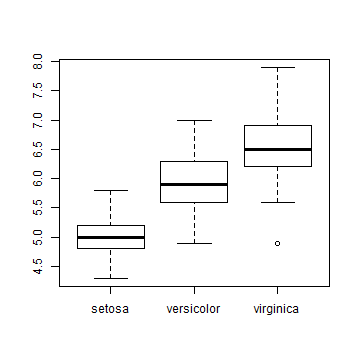If you wish to compare the medians of the boxplot, you can use the function `notch`. If the notches of two plots do not overlap, this is 'strong evidence' that the two medians differ (see ?boxplot)

``````
boxplot(iris\$Sepal.Length ~ iris\$Species, notch = T)
``````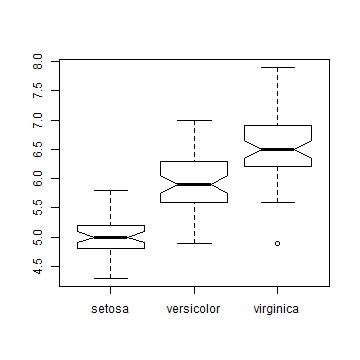You may have noticed that the y-axis labels are always orientated to be perpendicular to the axis. We can rotate all axis labels using `las`. Play around with different values.

``````boxplot(iris\$Sepal.Length ~ iris\$Species, notch = T, las = 1)
``````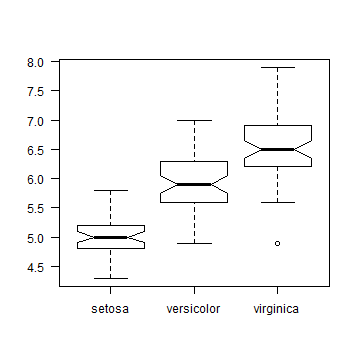Let's add in all the axis and plot labels:

``````
boxplot(iris\$Sepal.Length ~ iris\$Species,
notch = T,
las = 1,
xlab = "Species",
ylab = "Sepal Length",
main = "Sepal Length by Species in Iris")
``````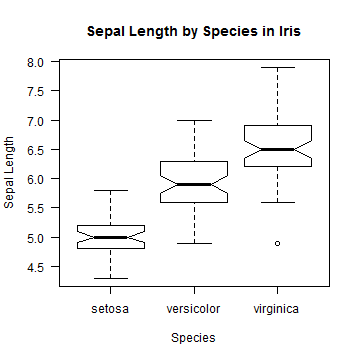Like we can change the size of the points in the scatterplot, we can change the size of the axis labels and titles. Let's start with `cex.lab`, which controls the axis titles:

``````
boxplot(iris\$Sepal.Length ~ iris\$Species,
notch = T,
las = 1,
xlab = "Species", ylab = "Sepal Length", main = "Sepal Length by Species in Iris",
cex.lab=1.5)
``````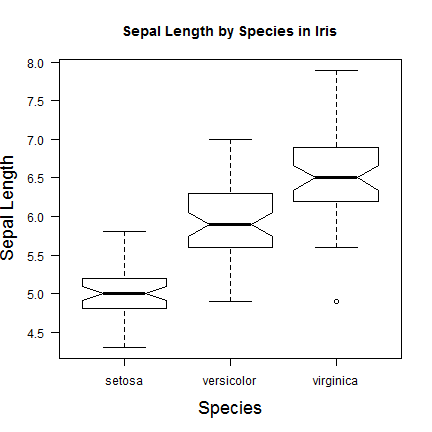Now we can add in “cex.axis” (changing the tickmark size) and “cex.main” (changing the plot title size)

``````
boxplot(iris\$Sepal.Length ~ iris\$Species, notch = T, las = 1,
xlab = "Species", ylab = "Sepal Length", main = "Sepal Length by Species in Iris",
cex.lab = 1.5,
cex.axis = 1.5,
cex.main = 2)
``````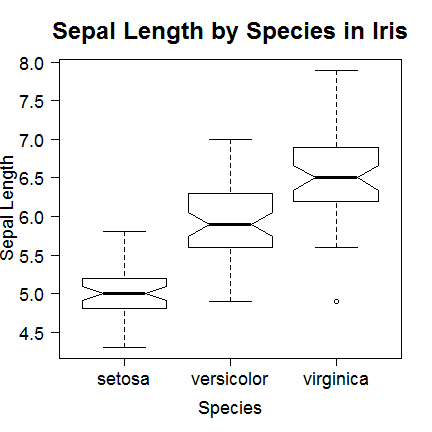As we discussed earlier, R automatically puts factors in alphabetical order. But perhaps we would prefer to list the iris species as virginica, versicolor and setosa. First lets look at the levels of iris:

``````data(iris)
levels(iris\$Species)
``````
``````##  "setosa"     "versicolor" "virginica"
``````

We reorder them with the following command:

``````
iris\$Species <- factor(iris\$Species, levels = c("virginica","versicolor","setosa"))
``````

Let's see that FINAL PLOT:

``````
boxplot(iris\$Sepal.Length ~ iris\$Species,              # x variable, y variable
notch = T,                                     # Draw notch
las = 1,                                       # Orientate the axis tick labels
xlab = "Species",                              # X-axis label
ylab = "Sepal Length",                         # Y-axis label
main = "Sepal Length by Species in Iris",      # Plot title
cex.lab = 1.5,                                 # Size of axis labels
cex.axis = 1.5,                                # Size of the tick mark labels
cex.main = 2)                                  # Size of the plot title
``````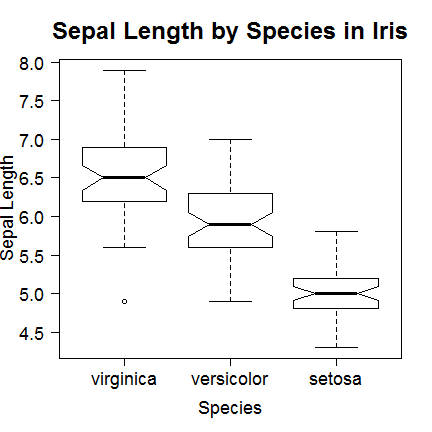# 5. Barplot with error bars using summary data

Ugh. I warn you - this will not be pretty.Let's create a new data frame with information on three populations of dragon in the UK:

``````dragons <- data.frame(
TalonLength = c(20.9, 58.3, 35.5),
SE = c(4.5, 6.3, 5.5),
Population = c("England", "Scotland", "Wales"))

dragons
``````
``````##   TalonLength  SE Population
## 1        20.9 4.5    England
## 2        58.3 6.3   Scotland
## 3        35.5 5.5      Wales
``````

Let's make our barplot.

``````
barplot(dragons\$Population, dragons\$TalonLength)
``````
``````## Error: 'height' must be a vector or a matrix
``````

No, this didn't work. It would be better to add Titles to the x-axis:

``````barplot(dragons\$TalonLength, names = dragons\$Population)
``````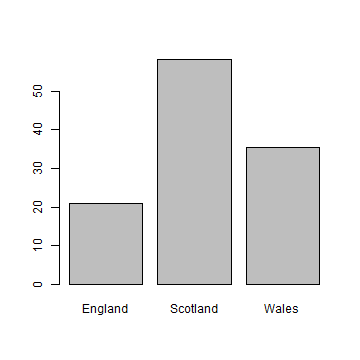Would a box look better around this plot?

``````barplot(dragons\$TalonLength, names = dragons\$Population)
box()
``````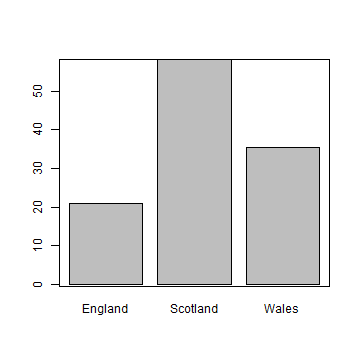Not really. Let's start again:

``````barplot(dragons\$TalonLength, names = dragons\$Population)
``````Let's reorder the columns by how beautiful the dragon habitat is (from best to worst). Naturally, this order is 'Scotland, Wales, England'.

``````levels(dragons\$Population)
``````
``````##  "England"  "Scotland" "Wales"
``````
``````
dragons\$Population <- factor(dragons\$Population, levels=c("Scotland","Wales","England"))

barplot(dragons\$TalonLength, names = dragons\$Population)
``````

No…. it's not working. I give up for now. What about error bars?

``````library(gplots)
``````
``````## Loading required package: gtools Loading required package: gdata gdata:
## Unable to locate valid perl interpreter gdata: gdata: read.xls() will be
## unable to read Excel XLS and XLSX files gdata: unless the 'perl=' argument
## is used to specify the location gdata: of a valid perl intrpreter. gdata:
## gdata: (To avoid display of this message in the future, please gdata:
## ensure perl is installed and available on the executable gdata: search
## path.) gdata: Unable to load perl libaries needed by read.xls() gdata: to
## support 'XLX' (Excel 97-2004) files.
##
## gdata: Unable to load perl libaries needed by read.xls() gdata: to support
## 'XLSX' (Excel 2007+) files.
##
## gdata: Run the function 'installXLSXsupport()' gdata: to automatically
## download and install the perl gdata: libaries needed to support Excel XLS
## and XLSX formats.
##
## Attaching package: 'gdata'
##
## The following object is masked from 'package:stats':
##
## nobs
##
## The following object is masked from 'package:utils':
##
## object.size
##
##
## Attaching package: 'gplots'
##
## The following object is masked from 'package:stats':
##
## lowess
``````
``````barplot(dragons\$TalonLength, names = dragons\$Population,
ylim=c(0,70),xlim=c(0,4),yaxs='i', xaxs='i',
main="Dragon Talon Length in the UK",
ylab="Mean Talon Length",
xlab="Country")
par(new=T)
plotCI (dragons\$TalonLength,
uiw = dragons\$SE, liw = dragons\$SE,
gap=0,sfrac=0.01,pch="",
ylim=c(0,70),
xlim=c(0.4,3.7),
yaxs='i', xaxs='i',axes=F,ylab="",xlab="")
``````
``````## Warning: "axes" is not a graphical parameter
``````
``````box()
``````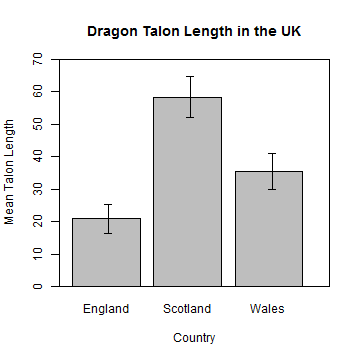Aaaaaaaaaaaargh!

FINAL PLOT

``````# Just do it in ggplot2!
``````

# Final words in base graphics

This is how I summed it up in the course: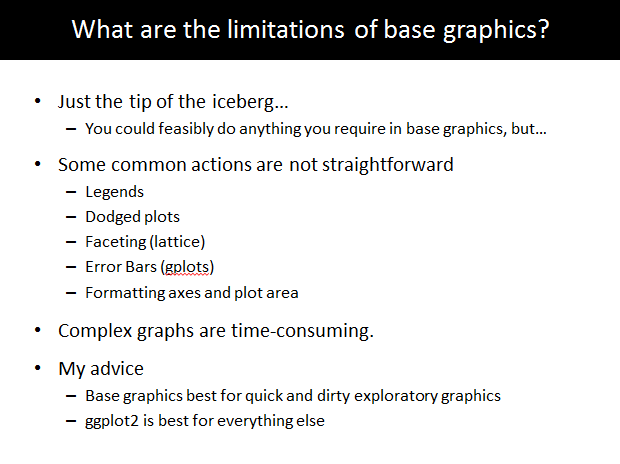# Extras!

Here is some code for some extra fun things in base graphics:

## 6. More than one plot in a window

``````

par(mfrow=c(1,2))      # number of rows, number of columns

plot(iris\$Sepal.Length, iris\$Petal.Length,        # x variable, y variable
col = iris\$Species,                          # colour by species
main = "Sepal vs Petal Length in Iris")      # plot title

plot(iris\$Sepal.Length, iris\$Sepal.Width,         # x variable, y variable
col = iris\$Species,                          # colour by species
main = "Sepal Length vs Width in Iris")      # plot title
``````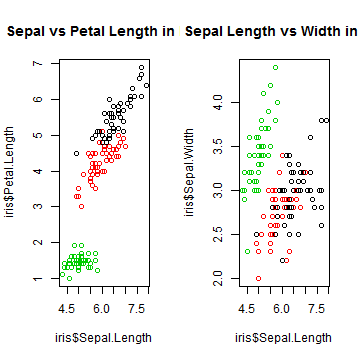``````
par(mfrow=c(1,1))     # sets the plot window back to normal

# OR

dev.off()      # But this will clear your plot history.
``````
``````## null device
##           1
``````

## 7. Saving a Plot

``````# png

png("Sepal vs Petal Length in Iris.png", width = 500, height = 500, res = 72)

plot(iris\$Sepal.Length, iris\$Petal.Length,
col = iris\$Species,
main = "Sepal vs Petal Length in Iris")

dev.off()
``````
``````## pdf
##   2
``````
``````

# pdf

pdf("Sepal vs Petal Length in Iris.pdf")

plot(iris\$Sepal.Length, iris\$Petal.Length,
col = iris\$Species,
main = "Sepal vs Petal Length in Iris")

dev.off()
``````
``````## pdf
##   2
``````